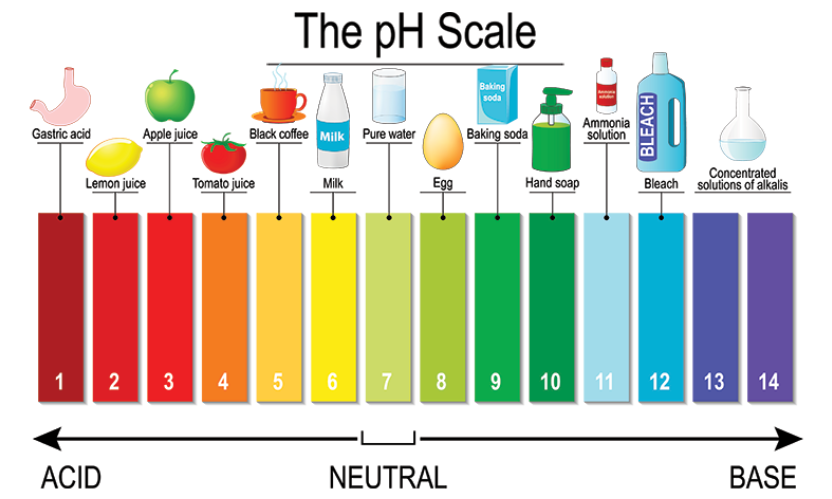# Identify the acid and base for ammonium chloride salt. What would be the nature of this salt? Mention the pH range of this salt.

Acid + Base -> Salt (NH4Cl) + Water
$HCl\ +\ NH_{4} OH\ \rightarrow \ NH_{4} Cl\ +\ H_{2} O$

From the above equation, we see that
Acid: Hydrochloric acid (HCl)
Base: Ammonium hydroxide (NH4OH)
Nature of salt: Acidic

Salts that produce H+ ions when dissolved in water making it acidic solutions are Acidic salts. These are produced by the reaction of strong acid and weak base. The pH is less than 7.

pH of salt: In between 4.5-5.5

(Refer image for better understanding)The pH of a solution is a measure of the molar concentration of hydrogen ions in the solution and as such is a measure of the acidity or basicity of the solution.

The letters pH stand for "power of hydrogen" and the numerical value for pH is just the negative of the power of 10 of the molar concentration of H+ ions.)

Updated on: 10-Oct-2022

55 Views